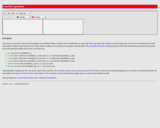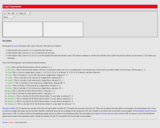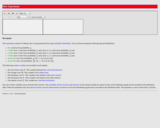Updating search results...

# 50 Results

View
Selected filters:
• University of Alabama in HuntsvilleUnrestricted Use
CC BY
Rating
0.0 stars

This resource consists of a Java applet and expository text. The applet is a simulation of the ballot experiment: The votes in an election are randomly counted. The event of interest is that the winning candidate is always ahead in the vote count.

Subject:
Mathematics
Probability and Statistics
Material Type:
Activity/Lab
Interactive
Simulation
Provider:
University of Alabama in Huntsville
Provider Set:
Virtual Laboratories in Probability and Statistics
Author:
Kyle Siegrist
05/04/2022Unrestricted Use
CC BY
Rating
0.0 stars

This resource consists of a Java applet and expository text. The applet is a simulation of Bertrand's experiment: a random chord on a circle The event of interest is whether the length of the chord is larger than the length of the inscribed equilateral triangle. Three models for generating the random chord can be used.

Subject:
Mathematics
Probability and Statistics
Material Type:
Activity/Lab
Interactive
Simulation
Provider:
University of Alabama in Huntsville
Provider Set:
Virtual Laboratories in Probability and Statistics
Author:
Kyle Siegrist
05/04/2022Unrestricted Use
CC BY
Rating
0.0 stars

This resource consists of a Java applet and expository text. The applet illustrates Bayesian estimation of the probability of heads for a coin. The prior beta distribution, true probability of heads, and the sample size can be specified. The applet shows the posterior beta distribution.

Subject:
Mathematics
Probability and Statistics
Material Type:
Activity/Lab
Interactive
Simulation
Provider:
University of Alabama in Huntsville
Provider Set:
Virtual Laboratories in Probability and Statistics
Author:
Kyle Siegrist
05/04/2022Unrestricted Use
CC BY
Rating
0.0 stars

This resource consists of a Java applet and expository text. The applet simulates a random sample from a beta distribution, and computes standard point estimates of the left and right parameters. The bias and mean square error are also computed.

Subject:
Mathematics
Probability and Statistics
Material Type:
Activity/Lab
Interactive
Simulation
Provider:
University of Alabama in Huntsville
Provider Set:
Virtual Laboratories in Probability and Statistics
Author:
Kyle Siegrist
05/04/2022Unrestricted Use
CC BY
Rating
0.0 stars

This resource consist of a Java applet and expository text. The applet simulates Bernoulli trials in terms of coin tosses. The random variables of interest are the number of heads and the proportion of heads. The number of coins and the probability of heads can be varied. The applet illustrates the law of large numbers and the central limit theorem.

Subject:
Mathematics
Probability and Statistics
Material Type:
Activity/Lab
Interactive
Simulation
Provider:
University of Alabama in Huntsville
Provider Set:
Virtual Laboratories in Probability and Statistics
Author:
Kyle Siegrist
05/04/2022Unrestricted Use
CC BY
Rating
0.0 stars

This resource consists of a Java applet and expository text. The applet simulates Bernoulli trials in terms of random points on a timeline. The random variables of interest are the number of successes and the proportion of successes. The number of trials and the probability of success can be varied. This applet illustrates the law of large numbers, the central limit theorem, and the binomial distribution.

Subject:
Mathematics
Probability and Statistics
Material Type:
Activity/Lab
Interactive
Simulation
Provider:
University of Alabama in Huntsville
Provider Set:
Virtual Laboratories in Probability and Statistics
Author:
Kyle Siegrist
05/04/2022Unrestricted Use
CC BY
Rating
0.0 stars

This resource consists of a Java applet and expository text. The applet is a simulation of the birthday experiment: a sample of size n is chose at random and with replacement from the first m positive integers. The random variable of interest is the number of distinct sample values. The event of interest is that all sample values are distinct.

Subject:
Mathematics
Probability and Statistics
Material Type:
Activity/Lab
Interactive
Simulation
Provider:
University of Alabama in Huntsville
Provider Set:
Virtual Laboratories in Probability and Statistics
Author:
Kyle Siegrist
05/04/2022Unrestricted Use
CC BY
Rating
0.0 stars

This resource consists of a Java applet and expository text. The applet simulates the bivariate normal distribution. The means are set at 0, but the standard deviations and the correlation can be varied. Simulated points from the distribution are shown as dots in a scatterplot.

Subject:
Mathematics
Probability and Statistics
Material Type:
Activity/Lab
Interactive
Simulation
Provider:
University of Alabama in Huntsville
Provider Set:
Virtual Laboratories in Probability and Statistics
Author:
Kyle Siegrist
05/04/2022Unrestricted Use
CC BY
Rating
0.0 stars

This resource consists of a Java applet and expository text. The Java applet illustrates the bivariate uniform distribution on three types of regions: a square, a circle, and a triangle. Simulated points from the distribution are shown as dots in a scatterplot.

Subject:
Mathematics
Probability and Statistics
Material Type:
Activity/Lab
Interactive
Simulation
Provider:
University of Alabama in Huntsville
Provider Set:
Virtual Laboratories in Probability and Statistics
Author:
Kyle Siegrist
05/04/2022Unrestricted Use
CC BY
Rating
0.0 stars

This resource consists of a Java applet and expository text. The applet simulates Buffon's coin experiment. The radius of the coin can be varied. The applet illustrates a random experiment, the sample space, random variables, events, probability, and relative frequency.

Subject:
Mathematics
Probability and Statistics
Material Type:
Activity/Lab
Interactive
Simulation
Provider:
University of Alabama in Huntsville
Provider Set:
Virtual Laboratories in Probability and Statistics
Author:
Kyle Siegrist
05/04/2022Unrestricted Use
CC BY
Rating
0.0 stars

This resource consists of a Java applet and expository text. The applet simulates Buffon's needle experiment and the corresponding approximation of pi. The event of interest is that the needle crosses a crack. The length of the needle can be varied. The applet illustrates a random experiment, the sample space, random variables, probability, and relative frequency.

Subject:
Mathematics
Probability and Statistics
Material Type:
Activity/Lab
Interactive
Simulation
Provider:
University of Alabama in Huntsville
Provider Set:
Virtual Laboratories in Probability and Statistics
Author:
Kyle Siegrist
05/04/2022Unrestricted Use
CC BY
Rating
0.0 stars

This resource consists of a Java applet and expository text. The applet is a simulation of drawing n cards from a standard deck. The parameter n can be varied.

Subject:
Mathematics
Probability and Statistics
Material Type:
Activity/Lab
Interactive
Simulation
Provider:
University of Alabama in Huntsville
Provider Set:
Virtual Laboratories in Probability and Statistics
Author:
Kyle Siegrist
05/04/2022Unrestricted Use
CC BY
Rating
0.0 stars

This resource consists of a Java applet and expository text. The applet simulates the chuck-a-luck experiment of rolling 3 fair dice. The random variable of interest is the net profit of the player.

Subject:
Mathematics
Probability and Statistics
Material Type:
Activity/Lab
Interactive
Simulation
Provider:
University of Alabama in Huntsville
Provider Set:
Virtual Laboratories in Probability and Statistics
Author:
Kyle Siegrist
05/04/2022Unrestricted Use
CC BY
Rating
0.0 stars

This resource consists of a Java applet and expository text. The applet is a simulation of the experiment that consists of tossing a coin and then rolling either a red die or a green die, depending on the outcome of the coin toss. The probability of heads and the distributions of the two dice can be specified. The applet illustrates a two-stage experiment.

Subject:
Mathematics
Probability and Statistics
Material Type:
Activity/Lab
Interactive
Simulation
Provider:
University of Alabama in Huntsville
Provider Set:
Virtual Laboratories in Probability and Statistics
Author:
Kyle Siegrist
05/04/2022Unrestricted Use
CC BY
Rating
0.0 stars

This resource consists of a Java applet and expository text. The applet simulates coin tosses. The number of coins and the probability of heads can be varied.

Subject:
Mathematics
Probability and Statistics
Material Type:
Activity/Lab
Simulation
Provider:
University of Alabama in Huntsville
Provider Set:
Virtual Laboratories in Probability and Statistics
Author:
Kyle Siegrist
01/18/2006Unrestricted Use
CC BY
Rating
0.0 stars

This resource consists of a Java applet and expository text. The applet is a simulation of the coupon collector problem, which consists of sampling from the first m positive integers, with replacement, until k distinct values are obtained. The parameters m and k can be varied. The random variable of interest is the size of the sample.

Subject:
Mathematics
Probability and Statistics
Material Type:
Activity/Lab
Interactive
Simulation
Provider:
University of Alabama in Huntsville
Provider Set:
Virtual Laboratories in Probability and Statistics
Author:
Kyle Siegrist
05/04/2022Unrestricted Use
CC BY
Rating
0.0 stars

This resource consists of a Java applet and expository text. The applet is a simulation of the standard casino craps game. The random variables of interest are the winning under various bets.

Subject:
Mathematics
Probability and Statistics
Material Type:
Activity/Lab
Interactive
Simulation
Provider:
University of Alabama in Huntsville
Provider Set:
Virtual Laboratories in Probability and Statistics
Author:
Kyle Siegrist
05/04/2022Unrestricted Use
CC BY
Rating
0.0 stars

This resource consists of a Java applet and expository text. The applet simulates rolling n dice. The random variables of interest are the sum of the scores, the maximum score, the minimum score, and the number of aces. The number of dice and the probability distribution that governs the dice can be specified. The applet illustrates various distributions and the central limit theorem.

Subject:
Mathematics
Probability and Statistics
Material Type:
Activity/Lab
Interactive
Simulation
Provider:
University of Alabama in Huntsville
Provider Set:
Virtual Laboratories in Probability and Statistics
Author:
Kyle Siegrist
05/04/2022Unrestricted Use
CC BY
Rating
0.0 stars

This resource consists of a Java applet and expository text. The applet simulates the rolling of n dice. The number of dice and the probability distribution that governs the dice can be specified.

Subject:
Mathematics
Probability and Statistics
Material Type:
Activity/Lab
Interactive
Simulation
Provider:
University of Alabama in Huntsville
Provider Set:
Virtual Laboratories in Probability and Statistics
Author:
Kyle Siegrist
05/04/2022Unrestricted Use
CC BY
Rating
0.0 stars

This resource consists of a Java applet and expository text. The applet simulates the experiment of rolling a die and then tossing a coin the number of times shown on the die. The die distribution and the probability of heads can be specified. The applet illustrates a two-stage experiment.

Subject:
Mathematics
Probability and Statistics
Material Type:
Activity/Lab
Interactive
Simulation
Provider:
University of Alabama in Huntsville
Provider Set:
Virtual Laboratories in Probability and Statistics
Author:
Kyle Siegrist